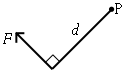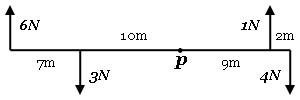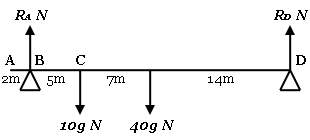# Moments

• Moments are about the turning effect produced by a force around a pivot point. The further away the force is to the pivot point, the greater the turning effect. As this is linear, the moment of a force about a point can calculated as the product of the magnitude of the force and the distance from the pivot point.
Moment = F × d…
• If the distance isn’t directly given, it can be worked out with some trigonometry magic.

• The overall turning effect on a body can be calculated as the sum of all the moments about a point choosing clockwise or anti-clockwise as a positive direction.…
• In the example above: 6×17 + 4×11 - (3×10 + 1×9) = 107 Nm Clockwise

• Problems relating to bodies in equilibrium can be solved by equating the clockwise and anti-clockwise moments about a point, given that the body will not be moving. It can also sometimes be helpful to resolve the forces horizontally, especially in scenarios where there is a rod resting on supports.

• A uniform rod can be considered as having its weight acting from its centre.…
Written by Sam Adam-Day.